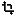# Geometry conversion

This category contains 10 nodes.

##### Filter geometry by type

This node filters geometries based on a given type.

##### Geometries to multi-geometries

This node returns an aggregated geometry from a collection of geometries.

##### Line endpoints

This nodes finds start and end points of a line geometry

##### Line merge

This node merges a collection of lines into a single line

##### Line to polygon

Converts linestring or multilinestring to polygon

##### Multi-geometry to geometries

This node converts a multi geometry to a list of geometries.

##### Polygon to line

This nodes finds lines of a polygon geometry

##### Snap to grid

This node snaps all points of the input geometry to a regular grid

##### Transform

This node transforms geometry to a different coordinate reference system.

##### Vertices to points

Converts the vertices of geometry to points